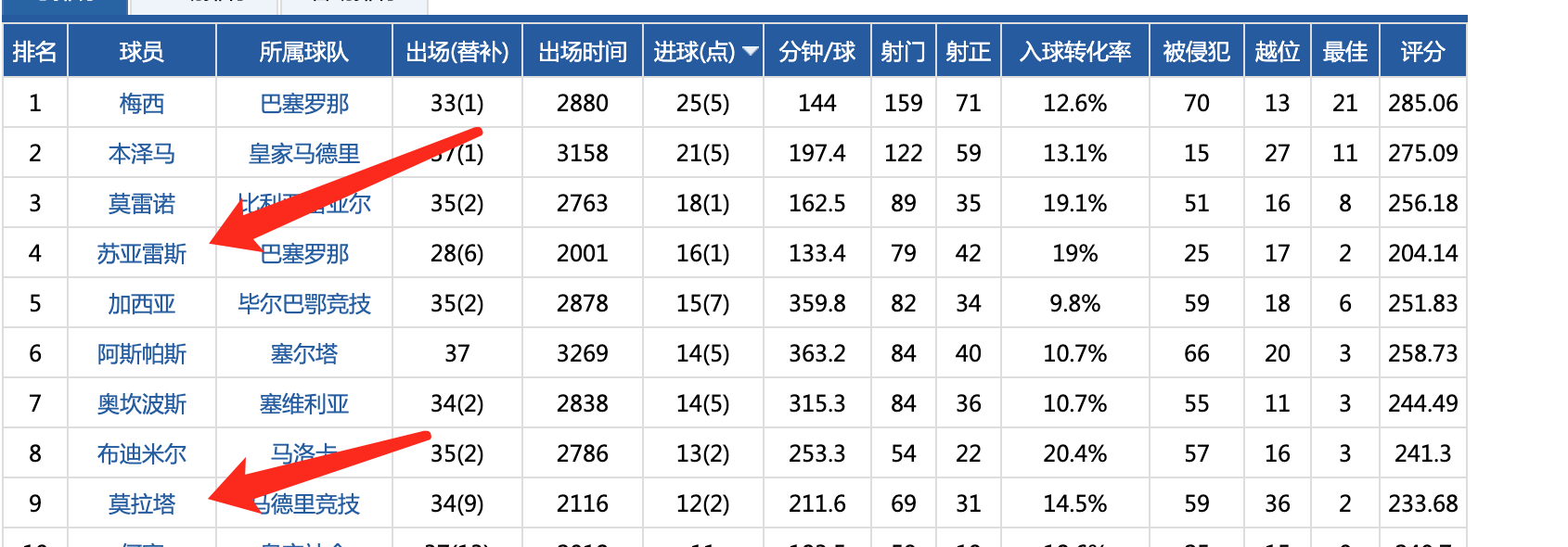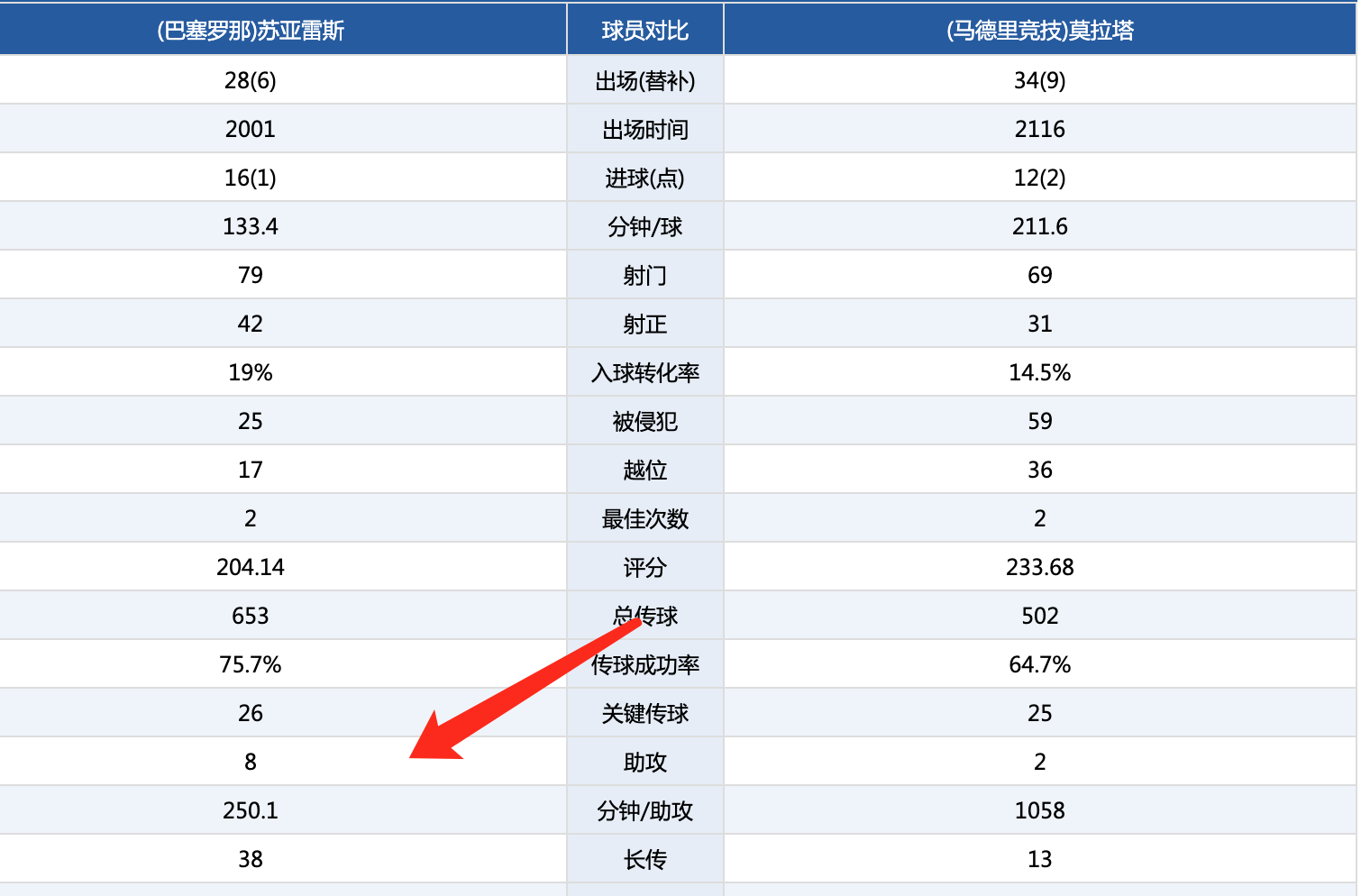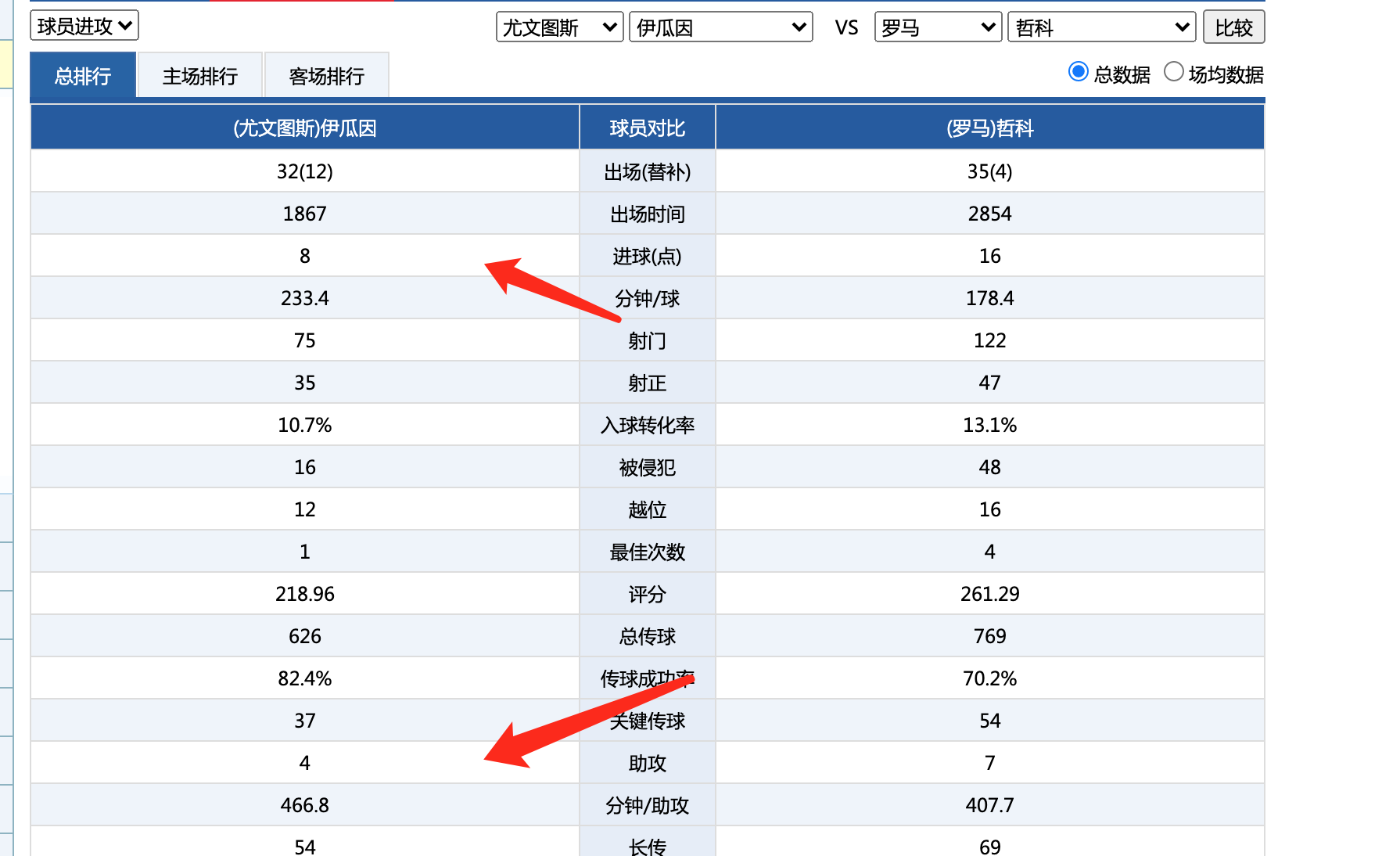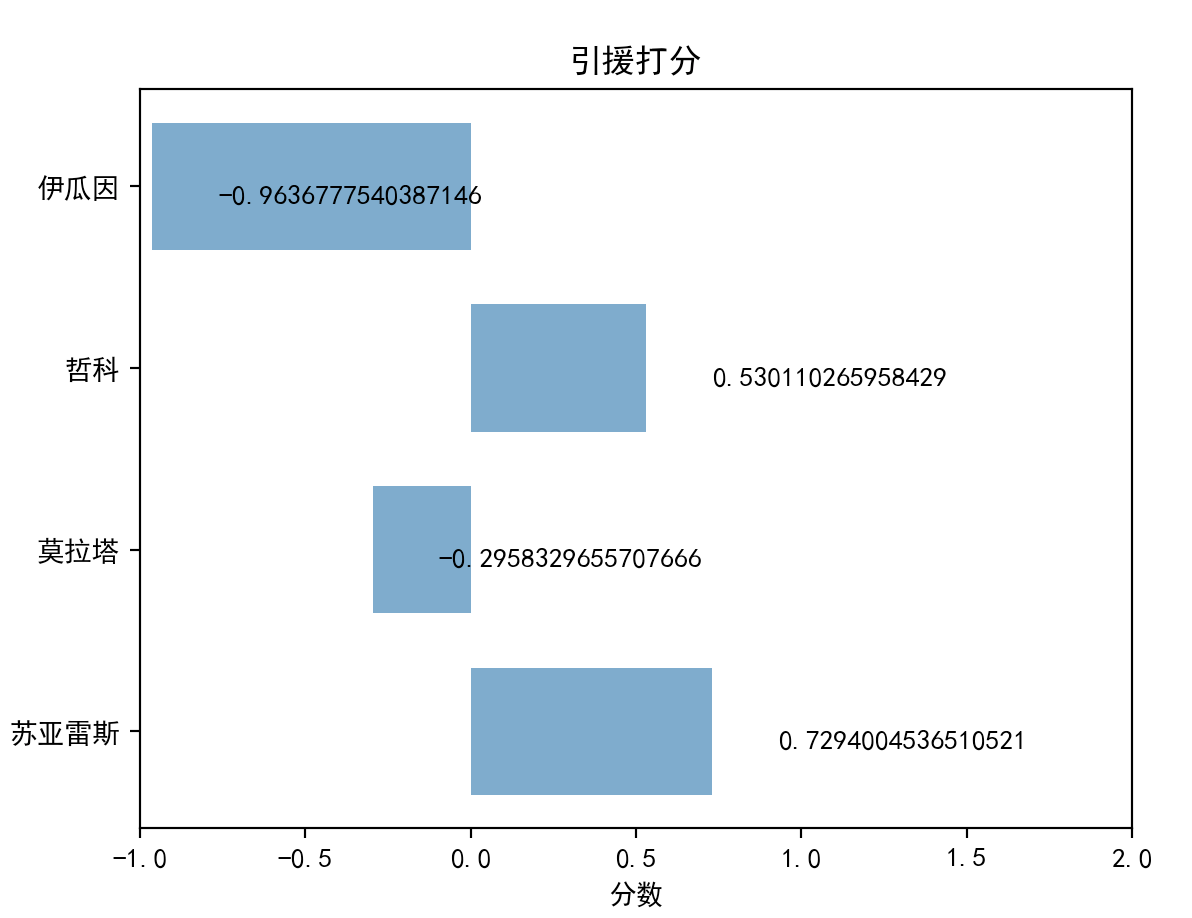# 鸟枪换炮，利用 python3 对球员做大数据降维 (因子分析得分)，为 C 罗找到合格僚机import pandas as pd  import numpy as np  from pandas import DataFrame,Series    #构建数据集  mydata = {         '进球':[16,12,16,8],         '进球转化率':[19,14,13,10],         '助攻':[8,2,7,4],  }  data = DataFrame(mydata)    data.index=['苏亚雷斯','莫拉塔','哲科','伊瓜因']    print(data)

进球  进球转化率  助攻  苏亚雷斯  16     19   8  莫拉塔   12     14   2  哲科    16     13   7  伊瓜因    8     10   4

from factor_analyzer import FactorAnalyzer, Rotator    fa = FactorAnalyzer(rotation=None)  fa.fit(data)print(fa.loadings_)

rotator = Rotator()  print("旋转后矩阵:\n", rotator.fit_transform(fa.loadings_))

print(fa.get_communalities())

print(fa.get_eigenvalues())

def F(factors):      return sum(factors*fa.get_factor_variance())

scores = []  for i in range(len(fa.transform(data))):      new = F(fa.transform(data)[i])      scores.append(new)    print(scores)

[0.7294004536510521, -0.2958329655707666, 0.530110265958429, -0.9636777540387146]

data['综合打分'] = scoresprint(data)

进球  进球转化率  助攻      综合打分  苏亚雷斯  16     19   8  0.729400  莫拉塔   12     14   2 -0.295833  哲科    16     13   7  0.530110  伊瓜因    8     10   4 -0.963678

data = data.sort_values(by='综合打分',ascending=False)

进球  进球转化率  助攻      综合打分  苏亚雷斯  16     19   8  0.729400  哲科    16     13   7  0.530110  莫拉塔   12     14   2 -0.295833  伊瓜因    8     10   4 -0.963678

import matplotlib.pyplot as plt  import matplotlib    matplotlib.rcParams['font.sans-serif'] = ['SimHei']  matplotlib.rcParams['axes.unicode_minus'] = False      plt.barh(range(4), scores, height=0.7, color='steelblue', alpha=0.8)    plt.yticks(range(4), ['苏亚雷斯','莫拉塔','哲科','伊瓜因'])  plt.xlim(-1,2)  plt.xlabel("分数")  plt.title("引援打分")  for x, y in enumerate(scores):      plt.text(y + 0.2, x - 0.1, '%s' % y)  plt.show()## 评论